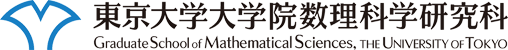このページに含まれる映像は自由に視聴することができますが、許可無くコピー・配布などの行為を禁止します。

# 数理談話会

### 講演者

Carlos Simpson 氏 (CNRS, University of Nice)

### 講演題目

Differential equations and the topology of algebraic varieties

### 講演概要

The study of the topology of complex algebraic varieties makes use of differential equations in several different ways. The classical notion of variation of Hodge structure contains, on the one hand, the Gauss-Manin differential equations, on the other hand Hodge metric data which satisfy harmonic bundle equations. These two aspects persist in the study of arbitrary representations of the fundamental group. Combining them leads to a notion of ``Hodge structure'' on the space of representations. This can be extended to the higher homotopical structure of a variety, by using ideas of ``shape'' and nonabelian cohomology.